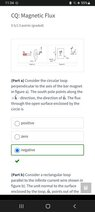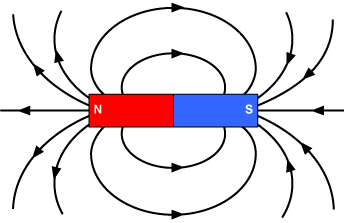# Flux from magnet (Why is the Flux not zero through the loop?)

Callumnc1
Homework Statement:
Relevant Equations:
For the problem attached,
Why is the magnetic Flux not zero through the loop?

Many thanks!

#### Attachments

•Screenshot_20230130-113420_Chrome.jpg
20.7 KB · Views: 12

Gold Member
Here‘s a picture of the field lines. Why do you think the answer is zero?•Callumnc1
Callumnc1
Here‘s a picture of the field lines. Why do you think the answer is zero?

View attachment 321439
Thanks for your reply @Frabjous! because the number of field lines going into the loop is equal to the number going out.

Homework Helper
This is an open surface. There is no in and out. These apply only to closed surfaces. You should review the definition of flux through a surface. According to your idea, the flux would be always zero.

•Callumnc1
Gold Member
The flux is a measure of the net amount going through a surface. What equation are you using for flux?

•Callumnc1
Callumnc1
The flux is a measure of the net amount going through a surface. What equation are you using for flux?
Thank you for your replies @nasu and @Frabjous ! The equation for magnetic flux is ##\phi = BA\cos\theta ##

Gold Member
What is the sign of cosθ for the surface of interest? Hint: the normal to the surface is pointing to the right. The B field is pointing to the left.

Last edited:
•Callumnc1
Callumnc1
What is the sign of cosθ for the surface of interest? Hint: the normal to the surface is pointing to the right. The B field is pointing to the left.
Thanks for your reply @Frabjous ! I think the direction of the B-field relative to the surface normal varies along the surface.

Many thanks!

Gold Member
Yes it varies, but it is all the same sign. What is the sign?

•Callumnc1
Callumnc1
Yes it varies, but it is all the same sign. What is the sign?
Thanks for your reply @Frabjous ! I think the sign is negative because ##\theta > 90 ## so ##\cos\theta < 0 ##

Thanks again, I see why the magnetic flux is negative now.

•Frabjous